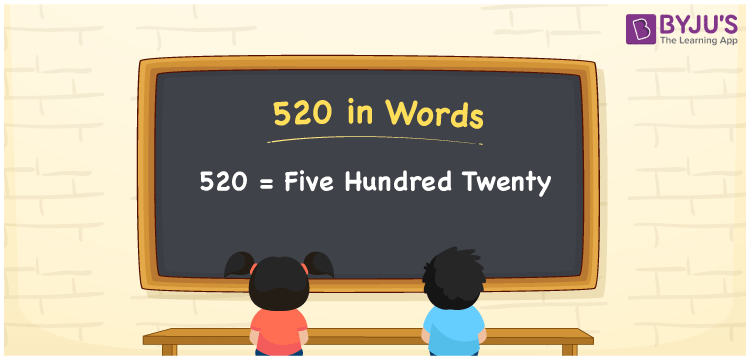# 520 in Words

520 in words is written as Five Hundred Twenty. For example, if you bought two pairs of socks worth Rs. 520, then you can say, “I bought two pairs of socks worth Rupees Five Hundred Twenty”. 520 is a cardinal number as it represents a specific quantity. Learn about the conversion of the number 520 in word form in this article.

 520 in Words Five Hundred Twenty Five Hundred Twenty in numerical form 520

## 520 in English Words

Generally, in Mathematics, we write the numbers in words with the help of the English alphabet. Hence, we can spell and write the number 520 in English as Five Hundred Twenty.## How to Write 520 in Words?

We can represent the number 520 in words using a place value chart. First, determine the position of each digit in the given number before writing its number name. The following table is the place value chart for the number 520.

 Hundreds Tens Ones 5 2 0

Therefore, we can write the expanded form as:

5 x Hundred + 2 x Ten + 0 x One

= 5 x 100 + 2 x 10 + 0 x 1

= 500 + 20 + 0

= 500 + 20

= 520

= Five Hundred Twenty

Hence, 520 in words is written as Five Hundred Twenty

Interesting way of writing 520 in words

5 = Five

52 = Fifty-Two

520 = Five Hundred Twenty

Thus, the word form of the number 520 is Five Hundred Twenty

520 is a natural number that precedes 521 and succeeds 519

• 520 in words – Five Hundred Twenty
• Is 520 an odd number? – No
• Is 520 an even number? – Yes
• Is 520 a perfect square number? – No
• Is 520 a perfect cube number? – No
• Is 520 a prime number? – No
• Is 520 a composite number? – Yes

## Frequently Asked Questions on 520 in Words

Q1

### How to write 520 in words?

520 in words is written as Five Hundred Twenty.
Q2

### What is the value of 520 + 20 in words?

520 + 20 = 540 i.e., Five Hundred Forty in words.
Q3

### 520 is a composite number. True or False.

True, the number 520 is a composite number.